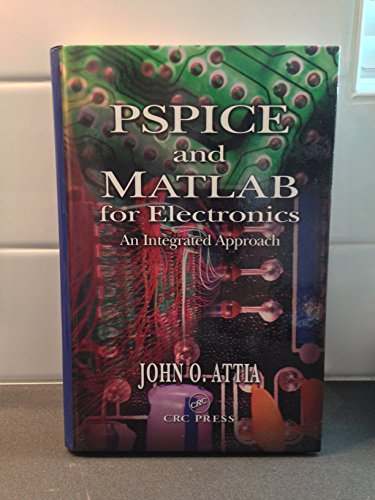# PSPICE and MATLAB for Electronics by John Okyere Attia (Prairie View A&M University, Texas, USA)

In Stock
\$16.99
+10% GST
The PSPICE and MATLAB software packages make a powerful combination for electronic circuit analysis, with PSPICE providing circuit analysis functions and MATLAB performing device parameters calculations, curve fitting, numerical functions, and plots. This book introduces readers to both of these industry-standard tools.
Only 1 left

## PSPICE and MATLAB for Electronics Summary

### PSPICE and MATLAB for Electronics: An Integrated Approach by John Okyere Attia (Prairie View A&M University, Texas, USA)

PSPICE has circuit simulation features unmatched by any other scientific software. MATLAB's capabilities for matrix computations, plotting, data processing, and analysis are well established throughout the world. Together, these two software packages form a powerful, full-function toolbox for electronic circuit analysis.

PSPICE and MATLAB for Electronics offers the first integrated presentation of both of these software packages. It provides a PSPICE primer, a MATLAB primer, and an in-depth treatment of their combined power for solving electronics problems, particularly those associated with diodes, op-amps, and transistor circuits. The author takes a practical approach, provides a multitude of examples, and encourages readers to put what they've learned into practice through the many exercises provided in each chapter. All of the PSPICE netlists and MATLAB m-files used in the examples are available on the Internet at www.crcpress.com.

Anyone working or aspiring to work in electronics needs a familiarity with these products, and learning to use them together offers more than the sum of their advantages. Use PSPICE for circuit analysis, use MATLAB for calculating device parameters, curve fitting, numerical functions, and plots, and use PSPICE and MATLAB for Electronics to learn how they can work in tandem to effectively and efficiently explore device characteristics and analyze circuits and systems.

### Why buy from World of BooksOur excellent value books literally don't cost the earthFree delivery in AustraliaEvery used book bought is one saved from landfill

## PSPICE and MATLAB for Electronics Reviews

"This textbook describes the combined abilities of PSPICE and MATLAB packages in solving integrated circuits and processing analysis dataThe heart of the book is the final three chapters, which describe the analysis of diode circuits, operational amplifiers, and transistor circuits, clearly exposing the power of the integrated usage of PSPICE and MATLAB. The most important accomplishment of the author lies in [chapters 5-7]. With the ease of using a high-level language, the book describes the combined power of PSPICE and MATLAB programming for analyzing diode, op-amps, and transistor circuitsThese chapters are bound to motivate any enthusiastic electronic engineer interested in simulation, modeling, and analysis of electronic circuits. This book has clearly exposed the strength of the integrated usage of PSPICE and MATLAB packages in solving electronic circuits. The numerous examples, both worked out and homework problems should provide the reader a good knowledge of the integrated usage of PSPICE and MATLAB packages" -K. Vasudevan, University of Bridgeport, Connecticut, USA "Each chapter contains a large number of worked PSPICE and MATLAB examplesHighly recommended as a useful addition for lower-division undergraduates through professionals, and two-year technical program students." - CHOICE, December 2002

PSPICE FUNDAMENTALS
Introduction
Elements Statements
Control Statements
DC Analysis (.DC)
Transient Analysis (.TRAN)
AC Analysis (.AC)
Printing Command (.PRINT)
Plotting Command (.PLOT)
Transfer Function COMMAND (.TF)
DC Sensitivity Analysis (.SENS)
Initial Conditions (.IC, UIC, .NODESET)
Temperature Analysis (.TEMP)
Probe Statement (.PROBE)
Device Model
Library File
Component Values (.PARAM, .STEP)
Function Definition (.FUNC, .INC)
Subcircuit (.SUBCKT, .ENDS)
Analog Behavioral Model
Monte Carlo Analysis (.MC)
Sensitivity and Worst Case Analysis (.WCASE)
Fourier Series (.FOUR)
MATLAB FUNDAMENTALS
MATLAB Basic Operations
Matrix Operations
Array Operations
Complex Numbers
The Colon Symbol
FOR Loops
IF Statements
Graph Functions
Input/Output Commands
MATLAB FUNCTIONS
M-Files
Mathematical Functions
Data Analysis Functions
Derivative Function (DIFF)
Curve Fitting (polyfit, polyval)
Polynomial Functions (roots, poly, polyval ,fzero)
Interfacing SPICE TO MATLAB
DIODE CIRCUITS
Diode
Rectification
Zener Diode Voltage Regulator
Peak Detector
Diode Limiters
OPERATIONAL AMPLIFIER
Inverting and Non-Inverting Configurations
Slew Rate and Full-Power Bandwidth
Active Filter Circuits
TRANSISTOR CHARACTERISTICS AND CIRCUITS
Characteristics of Bipolar Junction Transistors
MOSFET Characteristics
Biasing of BJT Circuits
MOSFET Bias Circuit
Frequency Response of Transistor Amplifiers
Feedback Amplifiers

Each chapter also includes a Bibliography and problems.

GOR010095535
PSPICE and MATLAB for Electronics: An Integrated Approach by John Okyere Attia (Prairie View A&M University, Texas, USA)
John Okyere Attia (Prairie View A&M University, Texas, USA)
VLSI Circuits
Used - Very Good
Hardback
Taylor & Francis Inc
2002-05-15
360
0849312639
9780849312632
N/A
Book picture is for illustrative purposes only, actual binding, cover or edition may vary.
This is a used book - there is no escaping the fact it has been read by someone else and it will show signs of wear and previous use. Overall we expect it to be in very good condition, but if you are not entirely satisfied please get in touch with us.